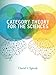Category Theory for the Sciences (The MIT Press)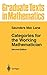Categories for the Working Mathematician (Graduate Texts in Mathematics, 5)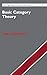Basic Category Theory (Cambridge Studies in Advanced Mathematics, Series Number 143)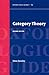Category Theory (Oxford Logic Guides, 52)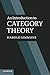An Introduction to Category Theory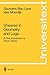Sheaves in Geometry and Logic: A First Introduction to Topos Theory (Universitext)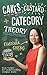Cakes, custard and category theory: easy recipes for understanding complex maths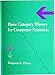Basic Category Theory for Computer Scientists (Foundations of Computing)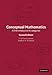Conceptual Mathematics: A First Introduction to Categories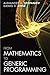From Mathematics to Generic Programming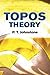Topos Theory (Dover Books on Mathematics)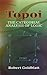Topoi: The Categorial Analysis of Logic (Dover Books on Mathematics)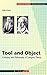Tool and Object: A History and Philosophy of Category Theory (Science Networks. Historical Studies, 32)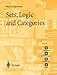Sets, Logic and Categories (Springer Undergraduate Mathematics Series)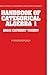Handbook of Categorical Algebra: Volume 1, Basic Category Theory (Encyclopedia of Mathematics and its Applications, Series Number 50)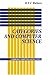Categories and Computer Science (Cambridge Computer Science Texts)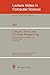Category Theory and Computer Programming: Tutorial and Workshop, Guildford, U.K., September 16 - 20, 1985. Proceedings (Lecture Notes in Computer Science, 240)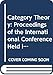Category Theory: Proceedings of the International Conference Held in Como, Italy, July 22-28, 1990 (Lectures Notes in Mathematics, Vol. 1488)